EngliSea > M > math > 60 Calculus

Whether differential or integral both concepts involve the idea that we can do something infinately many times and get a finite answer that is useful.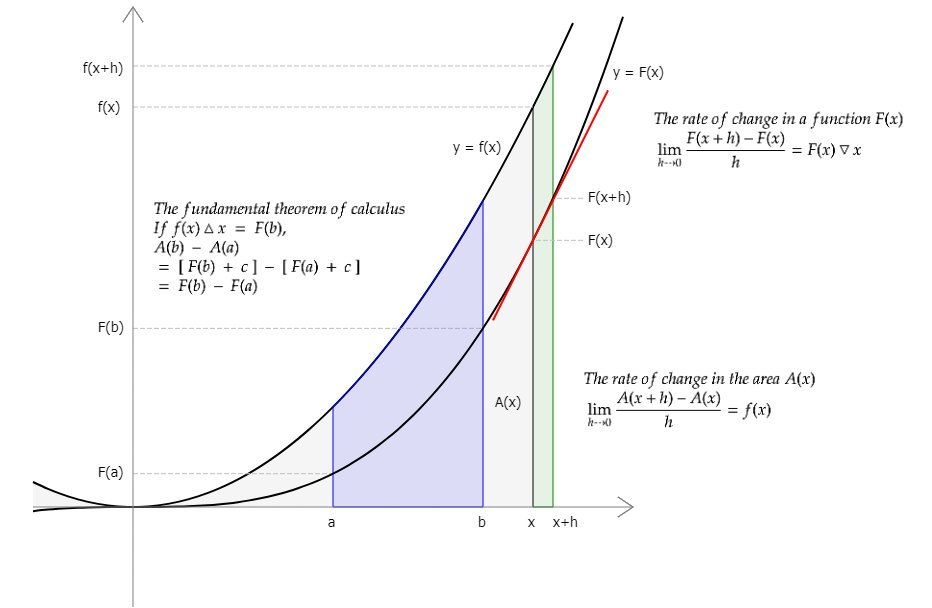Calculus summary
When x changes by h, the ratio of a change in F(x) to the change in x that caused it, is equal to (F(x+h)−F(x))/h. As h approaches 0, the limit of the ratio becomes a value. A function that maps x to this limit is called the derivative of F(x). ｢(F(x+h)− ...
365301 The Greeks, Newton and Leibniz
○ Many students quit math entirely when they get up to this point, mainly out of fear. ○ We will get to some of those in due time as well. ○ What challenge could possibly have necessitated the development of calculus?
5032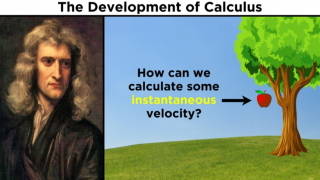01 The Greeks, Newton and Leibniz
Whether differential or integral both concepts involve the idea that we can do something infinately many times and get a finite answer that is useful.
9535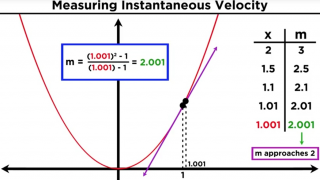03 Rates of Change
Galileo had already discovered some years prior that the distance traveled by a falling object is represented by a function of time. Newton wondered how one could calculate the velocity of the object at any particular instance during the fall.
5034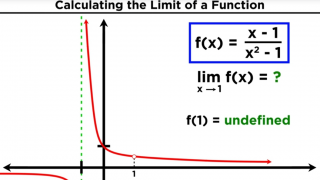04 Limits and Limit Laws in Calculus
Asymptote: a straight line approached by a given curve as one of the variables in the equation of the curve approaches infinity
5029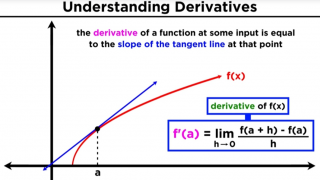05 What is a Derivative?
What is a Derivative? Deriving the Power Rule
5051

06 Power Rule proof
Definition of differentiation (x˄n)▽x = ｢((x+h)˄−x˄n)/h :h⨠0｣ Binomial Expansion (x+h)˄n = ｢n!/((n−k)!·k!)·x˄(n−k)·h˄k Σk=0,n｣ (x+h)˄n − x˄n = ｢n!/((n−k)!·k!)·x˄(n−k)·h˄k Σk=1,n｣ ((x+h)˄n−x˄n) / h = ｢n!/((n−k)!·k!)·x˄(n−k)·h˄(k−1) Σk=1,n｣ ｢((x+h)˄n−x˄ ...
9687

06 Product Rule proof
(f(x)·g(x))▽x = ｢(f(x+h)·g(x+h)−f(x)·g(x))/h :h⨠0｣ = ｢(f(x+h)·g(x+h)−f(x+h)·g(x)+f(x+h)·g(x)−f(x)·g(x))/h :h⨠0｣ ┅ −f(x+h)·g(x)+f(x+h)·g(x) inserted ┅ = ｢(f(x+h)·g(x+h)−f(x+h)·g(x))/h :h⨠0｣ + ｢(f(x+h)·g(x)−f(x)·g(x))/h :h⨠0｣ ┅ split up into two limits ┅ ...
9689

06 Quotient Rule proof
(f(x)/g(x))▽x = ｢(f(x+h)/g(x+h)−f(x)/g(x))/h :h⨠0｣ = ｢(f(x+h)·g(x)−f(x)·g(x+h))/(g(x+h)·g(x))/h :h⨠0｣ ➊ = ｢(f(x+h)·g(x)−f(x)·g(x+h))/h/(g(x+h)·g(x)) :h⨠0｣ = ｢(f(x+h)·g(x)−f(x)·g(x+h))/h :h⨠0｣ · ｢1/(g(x+h)·g(x)) :h⨠0｣ = ｢((f(x+h)−f(x))·g(x)−f(x)·(g(x+h)−g( ...
9692

06 Taylor and Maclaurin Series
○ Power Series ｢cₙ·x˄n Σn=0,∞｣ ○ Taylor Series ｢f(x)▽ⁿx｢a｣/n!·(x−a)˄n Σn=0,∞｣ ○ Maclaurin Series ｢f(x)▽ⁿx｢0｣/n!·x˄n Σn=0,∞｣ ┅ f(x)▽⁰x = c₀·(x−a)˄0 + c₁·(x−a)˄1 + c₂·(x−a)˄2 + c₃·(x−a)˄3 + ... = ｢cₙ·(x−a)˄n Σn=0,∞｣ f(x)▽⁰x｢a｣ = c₀ = c₀·0! c₀ = f(x)▽⁰x｢a｣/ ...
9697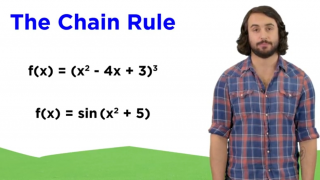08 Chain Rule
Derivatives of composite functions
9695

08 Chain Rule proof
Definition of differentiation f(g(x))▽x = ｢(f(g(x+h))−f(g(x)))/h :h⨠0｣ = ｢(f(g(x+h))−f(g(x)))/(g(x+h)−g(x))·(g(x+h)−g(x))/h :h⨠0｣ = ｢(f(g(x+h))−f(g(x)))/(g(x+h)−g(x)) :h⨠0｣·｢(g(x+h)−g(x))/h :h⨠0｣ ᐥ Let g(x+h)−g(x) = t, then ｢t :h⨠0｣ = 0 and g(x+h) = g(x) ...
9693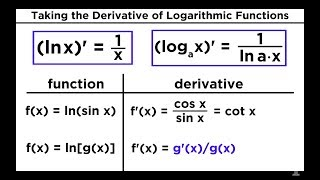09 Derivatives of Logarithmic and Exponential Functions
Derivatives of Logarithmic and Exponential Functions
3387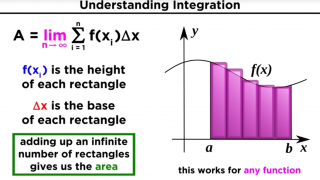17 What is Integration?
○ Finding the Area Under a Curve ○ tinker, articulation, solidify
512318 The fundamental theorem of calculus
If f is Riemann integrable on [a,b] and F(x) is the integral of f(t) from a to b then F is continuous on [a,b]. Furthermore, if f is continuous on [a,b] then F is differentiable on [a,b] and F' = f.
5193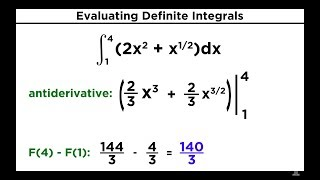19 Properties of Integrals and Evaluating Definite Integrals
Properties of Integrals and Evaluating Definite Integrals
3416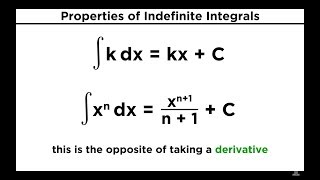20 Evaluating Indefinite Integrals
Evaluating Indefinite Integrals
3417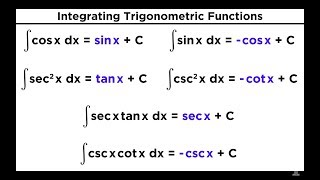21 Evaluating Integrals With Trigonometric Functions
Evaluating Integrals With Trigonometric Functions
3418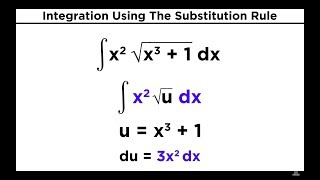22 Integration Using The Substitution Rule
Integration Using The Substitution Rule
3419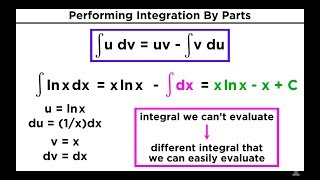23 Integration By Parts
Integration By Parts
3420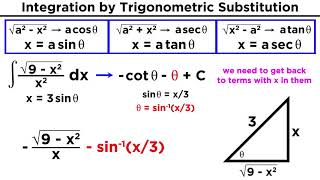24 Integration by Trigonometric Substitution
Integration by Trigonometric Substitution
3421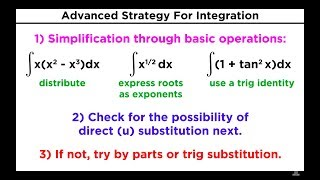25 Advanced Strategy for Integration in Calculus
Advanced Strategy for Integration in Calculus
3422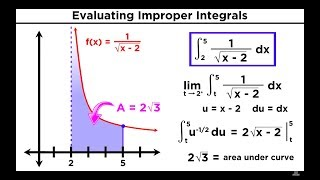26 Evaluating Improper Integrals
Evaluating Improper Integrals
3423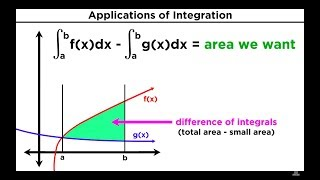27 Finding the Area Between Two Curves by Integration
Finding the Area Between Two Curves by Integration
3424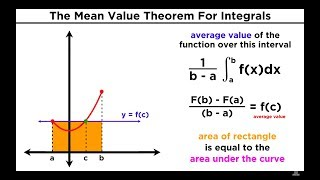30 The Mean Value Theorem For Integrals: Average Value of a Function
The Mean Value Theorem For Integrals: Average Value of a Function
3427-